# What is algebraic number theory?

A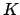is a finite algebraic extension of the rational numbers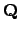. Every such extension can be represented as all polynomials in an algebraic number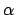: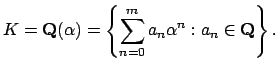Hereis a root of a polynomial with coefficients in. Note that tex2html_wrap_inline$Q(&alpha#alpha;)$ is non-canonically isomorphic to tex2html_wrap_inline$Q[x]/(f)$, where tex2html_wrap_inline$f$is the minimal polynomial of tex2html_wrap_inline$&alpha#alpha;$. The isomorphism is induced by the homomorphism tex2html_wrap_inline$Q[x]&rarr#to;Q(&alpha#alpha;)$ that sends tex2html_wrap_inline$x$ to tex2html_wrap_inline$&alpha#alpha;$, which has kernel tex2html_wrap_inline$(f)$. It is not canonical, since tex2html_wrap_inline$Q(&alpha#alpha;)$ could have nontrivial automorphisms. For example, if tex2html_wrap_inline$&alpha#alpha;=2$, then tex2html_wrap_inline$Q(2)$ is isomorphic as a field to tex2html_wrap_inline$Q(-2)$ via tex2html_wrap_inline$2&map#mapsto;-2$. There are two isomorphisms tex2html_wrap_inline$Q[x]/(x^2-2)&rarr#to;Q(2)$.

involves using techniques from (mostly commutative) algebra and finite group theory to gain a deeper understanding of number fields. The main objects that we study in algebraic number theory are number fields, rings of integers of number fields, unit groups, ideal class groups,norms, traces, discriminants, prime ideals, Hilbert and other class fields and associated reciprocity laws, zeta and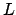-functions, and algorithms for computing each of the above.

Subsections
William Stein 2004-05-06